Main Content

# idfrd

Frequency response data or model

## Description

An `idfrd` object stores frequency response data over a range of frequency values. You can use an `idfrd` object in two ways. You can use the object as estimation data for estimating a time-domain or frequency-domain model, similarly to an `iddata` object. Or, you can use the object as a linear model, similarly to how you use an `idss` state-space model or any other identified linear model. Use the `idfrd` command to encapsulate frequency response data or to convert a linear time-domain or frequency-domain dynamic model into a frequency response model.

Commands that accept `iddata` objects, such as the model estimation command `ssest`, generally also accept `idfrd` objects. However, an `idfrd` object can contain data from only one experiment. It does not have the multiexperiment capability that an `iddata` object has.

Commands that accept identified linear models, such as the analysis and validation commands `compare`, `sim`, and `bode`, generally also accept `idfrd` models.

For a model of the form

`$y\left(t\right)=G\left(q\right)u\left(t\right)+H\left(q\right)e\left(t\right)$`

the transfer function estimate is $G\left({e}^{i\omega }\right)$ and the additive noise spectrum Φv at the output is

`${\Phi }_{v}\left(\omega \right)=\lambda T{|H\left(e{}^{i\omega T}\right)|}^{2}$`

Here, λ is the estimated variance of e(t) and T is the sample time.

For a continuous-time system, the noise spectrum is

`${\Phi }_{v}\left(\omega \right)=\lambda {|H\left(e{}^{i\omega }\right)|}^{2}$`

An `idfrd` object stores $G\left({e}^{i\omega }\right)$ and Φv.

## Creation

You can obtain an `idfrd` model in one of three ways.

• Create the model from frequency response data using the `idfrd` command. For example, create an `idfrd` model that encapsulates frequency response data taken at specific frequencies using the sample time `Ts`.

`sysfr = idfrd(ResponseData,Freq,Ts)`
For an example, see Create idfrd Object from Frequency Response Data.

• Estimate the model using a frequency response estimation command such as `spa`, using time-domain, frequency-domain, or frequency response data.

`sysfr = spa(data)`

For more information about frequency response estimation commands, see `spa`, `spafdr`, and `etfe`.

• Convert a linear model such as an `idss` model into an `idfrd` model by computing the frequency response of the model.

`sysfr = idfrd(sys)`
For an example of linear model conversion, see Convert Time-Domain Model to Frequency Response Model.

For information on functions you can use to extract information from or transform `idfrd` model objects, see Object Functions.

### Syntax

``sysfr = idfrd(ResponseData,Frequency,Ts)``
``sysfr = idfrd(___,Name,Value)``
``sysfr = idfrd(sys)``
``sysfr = idfrd(sys,Frequency)``
``sysfr = idfrd(sys,Frequency,FrequencyUnits)``

### Description

#### Create Frequency Response Object

example

````sysfr = idfrd(ResponseData,Frequency,Ts)` creates a discrete-time `idfrd` object that stores the frequency response `ResponseData` of a linear system at frequency values `Frequency`. `Ts` is the sample time. For a continuous-time system, set `Ts` to `0`.```
````sysfr = idfrd(___,Name,Value)` sets additional properties using one or more name-value arguments. Specify the name-value arguments after the first three arguments. For instance, to specify the frequency units as MHz, use ```sysfr = idfrd(ResponseData,Frequency,Ts,'FrequencyUnits','MHz')```. ```

#### Convert Linear Identified Model to Frequency Response Model

example

````sysfr = idfrd(sys)` converts a System Identification Toolbox™ or Control System Toolbox™ linear model to frequency response data at default frequencies, including the output noise spectra and spectra covariance.```
````sysfr = idfrd(sys,Frequency)` computes the frequency response at frequencies `Frequency`, where `Frequency` is expressed in radians/`TimeUnit`.```
````sysfr = idfrd(sys,Frequency,FrequencyUnits)` interprets frequencies in the `Frequency` vector in the units specified by `FrequencyUnit`.```

### Input Arguments

expand all

Linear dynamic system model, specified as a System Identification Toolbox or Control System Toolbox linear model.

## Properties

expand all

Frequency response data, specified as a 3-D array of complex numbers.

• For SISO systems, `ResponseData` is a vector of frequency response values at the frequency points specified in the `Frequency` property.

• For MIMO systems with Nu inputs and Ny outputs, `ResponseData` is an Ny-by-Nu-by-Nf array, where Nf is the number of frequency points.

`ResponseData(ky,ku,kf)` represents the frequency response from the input `ku` to the output `ky` at the frequency `Frequency(kf)`.

Frequency points corresponding to `ResponseData`, specified as a column vector that contains Nf points in the units specified by `FrequencyUnit`.

Units of the frequency vector in the `Frequency` property, specified as one of the following values:

• `'rad/TimeUnit'`

• `'cycles/TimeUnit'`

• `'rad/s'`

• `'Hz'`

• `'kHz'`

• `'MHz'`

• `'GHz'`

• `'rpm'`

The units `'rad/TimeUnit'` and `'cycles/TimeUnit'` are relative to the time units specified in the `TimeUnit` property.

Changing this property does not resample or convert the data. Modifying the property changes only the interpretation of the existing data. Use `chgTimeUnit` to convert the data to different frequency units.

Power spectra and cross spectra of the system output disturbances (noise), specified as a vector (single-output system) or a 3-D array of complex numbers (multiple-output system). For response data with Ny outputs and Nf frequency points, specify `SpectrumData` as an Ny-by-Ny-by-Nw array.

`SpectrumData(ky1,ky2,kf)` is the cross spectrum between the noise at output `ky1` and the noise at output `ky2` at the frequency `Frequency(kf)`. The power spectrum is the subset of the cross spectrum where `ky1` and `ky2` are equal.

Covariance of `SpectrumData`, specified as a 5-D array with dimensions Ny-by-Nu-by-Nf-by-2-by-2, where Ny is the number of outputs, Nu is the number of inputs, and Nf is the number of frequency points.

`CovarianceData(ky,ku,kf,:,:)` is the 2-by-2 covariance matrix of `SpectrumData(ky,ku,kf)`. The (1,1) element is the variance of the real part, the (2,2) element is the variance of the imaginary part, and the (1,2) and (2,1) elements are the covariance between the real and imaginary parts. `squeeze(CovarianceData(ky,ku,kf,:,:))` thus gives the covariance matrix of the corresponding response.

If you obtain `sysfr` by converting a model `sys`, the value of `CovarianceData` depends on how you obtained `sys`.

• If you obtained `sys` by identification, the software computes the estimated covariance for `sysfr` from the uncertainty information in `sys`. The software uses Gauss' approximation formula for this calculation for all model types, except grey-box models. For grey-box models (`idgrey`), the software applies numerical differentiation.

• If you created `sys` by using commands such as `idss`, `idtf`, `idproc`, `idgrey`, or `idpoly`, then the software sets `CovarianceData` for `sysfr` to `[]`.

Power spectra variance, specified as a vector (single-output system) or a 3-D array (multiple-output system). For response data with Ny outputs and Nw frequency points, specify `NoiseCovariance` as an Ny-by-Ny-by-Nw array. `NoiseCovariance(ky1,ky2,kw)` is the variance of the corresponding power spectrum.

To eliminate the influence of the noise component from the model, specify `NoiseCovariance` as `0`. With zero covariance, the predicted output is the same as the simulated output.

Intersample behavior of the input signal for transformations between discrete time and continuous time, specified as a character vector or as an Nu-by-1 cell array of character vectors, where Nu is the number of input channels. This property is meaningful only when you are estimating continuous-time models (sample time `Ts` > 0) from discrete-time data.

For each input channel, the possible values of `InterSample` are:

• `'zoh'` — Zero-order hold maintains a piecewise-constant input signal between samples.

• `'foh'` — First-order hold maintains a piecewise-linear input signal between samples.

• `'bl'` — Band-limited behavior specifies that the continuous-time input signal has zero power above the Nyquist frequency (`pi/sys.Ts` rad/s). This behavior typically occurs when the input signal is measured experimentally using an antialiasing filter and a sampler. Ideally, treat the data as continuous-time. That is, if the signals used for the estimation of the frequency response were subject to anti-aliasing filters, set `sys.Ts` to zero.

If you obtain `sysfr` by conversion of a model `sys`, then `InterSample` is equal to the `Intersample` property of the `iddata` object that you used to estimate `sys`.

For more information on this property, see Effect of Input Intersample Behavior on Continuous-Time Models.

Transport delays, specified as a numeric array containing a separate transport delay for each input-output pair.

For continuous-time systems, transport delays are expressed in the time unit stored in the `TimeUnit` property. For discrete-time systems, transport delays are expressed as integers denoting delays of a multiple of the sample time `Ts`.

For a MIMO system with Ny outputs and Nu inputs, set `IODelay` as an Ny-by-Nu array. Each entry of this array is a numerical value representing the transport delay for the corresponding input-output pair. You can set `IODelay` to a scalar value to apply the same delay to all input-output pairs.

Input delay for each input channel, specified as a scalar value or numeric vector. For continuous-time systems, specify input delays in the time unit stored in the `TimeUnit` property. For discrete-time systems, specify input delays in integer multiples of the sample time `Ts`. For example, setting `InputDelay` to `3` specifies a delay of three sample times.

For a system with Nu inputs, set `InputDelay` to an Nu-by-1 vector. Each entry of this vector is a numerical value that represents the input delay for the corresponding input channel.

You can also set `InputDelay` to a scalar value to apply the same delay to all channels.

For identified systems such as `idfrd`, `OutputDelay` is fixed to zero.

Sample time, specified as one of the following.

• Discrete-time model with a specified sampling time — a positive scalar representing the sampling period expressed in the unit specified by the `TimeUnit` property of the model

• Continuous-time model — `0`

• Discrete-time model with an unspecified sample time — `-1`

Changing this property does not discretize or resample the model. Use `c2d` and `d2c` to convert between continuous- and discrete-time representations. Use `d2d` to change the sample time of a discrete-time system.

Units for the time variable, the sample time `Ts`, and any time delays in the model, specified as one of the following values.

• `'nanoseconds'`

• `'microseconds'`

• `'milliseconds'`

• `'seconds'`

• `'minutes'`

• `'hours'`

• `'days'`

• `'weeks'`

• `'months'`

• `'years'`

Changing this property does not resample or convert the data. Modifying the property changes only the interpretation of the existing data. Use `chgTimeUnit` (Control System Toolbox) to convert data to different time units

Input channel names, specified as a character vector or cell array.

• Single-input model — Character vector. For example, `'controls'`.

• Multi-input model — Cell array of character vectors.

Alternatively, use automatic vector expansion to assign input names for multi-input models. For example, if `sys` is a two-input model, enter:

`sys.InputName = 'controls';`

The input names automatically expand to `{'controls(1)';'controls(2)'}`.

When you estimate a model using an `iddata` object `data`, the software automatically sets `InputName` to `data.InputName`.

You can use the shorthand notation `u` to refer to the `InputName` property. For example, `sys.u` is equivalent to `sys.InputName`.

You can use input channel names in several ways, including:

• To identify channels on model display and plots

• To extract subsystems of MIMO systems

• To specify connection points when interconnecting models

Input channel units, specified as a character vector or cell array:

• Single-input model — Character vector

• Multi-input Model — Cell array of character vectors

Use `InputUnit` to keep track of input signal units. `InputUnit` has no effect on system behavior.

Input channel groups, specified as a structure. The `InputGroup` property lets you divide the input channels of MIMO systems into groups so that you can refer to each group by name. In the `InputGroup` structure, set field names to the group names, and field values to the input channels belonging to each group.

For example, create input groups named `controls` and `noise` that include input channels 1 and 2 and channels 3 and 5, respectively.

```sys.InputGroup.controls = [1 2]; sys.InputGroup.noise = [3 5];```

You can then extract the subsystem from the `controls` inputs to all outputs using the following syntax:

`sys(:,'controls')`

Output channel names, specified as a character vector or cell array.

• Single-input model — Character vector. For example, `'measurements'`

• Multi-input model — Cell array of character vectors

Alternatively, use automatic vector expansion to assign output names for multi-output models. For example, if `sys` is a two-output model, enter:

`sys.OutputName = 'measurements';`

The output names automatically expand to `{'measurements(1)';'measurements(2)'}`.

When you estimate a model using an `iddata` object `data`, the software automatically sets `OutputName` to `data.OutputName`.

You can use the shorthand notation `y` to refer to the `OutputName` property. For example, `sys.y` is equivalent to `sys.OutputName`.

You can use output channel names in several ways, including:

• To identify channels on model display and plots

• To extract subsystems of MIMO systems

• To specify connection points when interconnecting models

Output channel units, specified as a character vector or cell array.

• Single-input model — Character vector. For example, `'seconds'`.

• Multi-input model — Cell array of character vectors.

Use `OutputUnit` to keep track of output signal units. `OutputUnit` has no effect on system behavior.

Output channel groups, specified as a structure. The `OutputGroup` property lets you divide the output channels of MIMO systems into groups and refer to each group by name. In the `OutputGroup` structure, set field names to the group names, and field values to the output channels belonging to each group.

For example, create output groups named `temperature` and `measurement` that include output channel 1, and channels 3 and 5, respectively.

```sys.OutputGroup.temperature = ; sys.OutputGroup.measurement = [3 5];```

You can then extract the subsystem from all inputs to the `measurement` outputs using the following syntax.

`sys('measurement',:)`

System name, specified as a character vector. For example, `'system_1'`.

Any text that you want to associate with the system, specified as a string or a cell array of character vectors. The property stores whichever data type you provide. For instance, if `sys1` and `sys2` are dynamic system models, you can set their `Notes` properties as follows.

```sys1.Notes = "sys1 has a string."; sys2.Notes = 'sys2 has a character vector.'; sys1.Notes sys2.Notes```
```ans = "sys1 has a string." ans = 'sys2 has a character vector.' ```

Data to associate with the system, specified as any MATLAB data type.

Sampling grid for model arrays, specified as a structure.

For arrays of identified linear (IDLTI) models that you derive by sampling one or more independent variables, this property tracks the variable values associated with each model. This information appears when you show or plot the model array. Use this information to trace results back to the independent variables.

Set the field names of the data structure to the names of the sampling variables. Set the field values to the sampled variable values associated with each model in the array. All sampling variables must be numeric and scalar valued, and all arrays of sampled values must match the dimensions of the model array.

For example, suppose that you collect data at various operating points of a system. You can identify a model for each operating point separately and then stack the results together into a single system array. You can tag the individual models in the array with information regarding the operating point.

```nominal_engine_rpm = [1000 5000 10000]; sys.SamplingGrid = struct('rpm', nominal_engine_rpm)```

Here, `sys` is an array containing three identified models obtained at 1000, 5000, and 10,000 rpm, respectively.

For model arrays that you generate by linearizing a Simulink® model at multiple parameter values or operating points, the software populates `SamplingGrid` automatically with the variable values that correspond to each entry in the array.

This property is read-only.

Summary report that contains information about the estimation options and results when the frequency-response model is obtained using estimation commands, such as `spa`, `spafdr`, and `etfe`. Use `Report` to query a model for how it was estimated, including its:

• Estimation method

• Estimation options

The contents of `Report` are irrelevant if the model was created by construction.

```f = logspace(-1,1,100); [mag,phase] = bode(idtf([1 .2],[1 2 1 1]),f); response = mag.*exp(1j*phase*pi/180); sysfr = idfrd(response,f,0.08); sysfr.Report.Method```
```ans = ''```

If you obtain the frequency-response model using estimation commands, the fields of `Report` contain information on the estimation data, options, and results.

```load iddata3; sysfr = spa(z3);sysfr.Report.Method```
```ans = SPA```

For more information on this property and how to use it, see the Output Arguments section of the corresponding estimation command reference page and Estimation Report.

## Object Functions

Many functions applicable to Dynamic System Models are also applicable to an `idfrd` model object. These functions are of three general types.

• Functions that operate on and return `idfrd` model objects enable you to convert and manipulate `idfrd` models.

• Functions that perform analytical and simulation functions on `idfrd` objects, such as `bode` and `sim`

• Functions that retrieve or interpret model information, such as `getcov`

Unlike other identified linear models, you cannot directly convert an `idfrd` model into another model type using commands such as `idss` or `idtf`. Instead, use the estimation command for the model you want, using the `idfrd` object as the estimation data. For instance, use `sys = ssest(sysfr,2)` to estimate a second-order state-space model from the frequency response data in `idfrd` model `sysfr`. For an example of using an `idfrd` object as estimation data, see Estimate Time-Domain Model Using Frequency Response Data.

The following lists contain a representative subset of the functions that you can use with `idss` models.

expand all

 `chgTimeUnit` Change time units of dynamic system `chgFreqUnit` Change frequency units of frequency-response data model `fselect` Select frequency points or range in FRD model `frdata` Access data for frequency response data (FRD) object `fcat` Concatenate FRD models along frequency dimension

expand all

 `bode` Bode plot of frequency response, or magnitude and phase data `spectrum` Plot or return output power spectrum of time series model or disturbance spectrum of linear input-output model

expand all

 `get` Access model property values `getcov` Parameter covariance of identified model

## Examples

collapse all

Create an `idfrd` object from frequency response data.

Load the magnitude data `AMP`, the phase data `PHA`, and the frequency vector `W`. Set sample time `Ts` to 0.1.

```load demofr AMP PHA W Ts = 0.1;```

Use the values of `AMP` and `PHA` to compute the complex-valued response `response`.

`response = AMP.*exp(1j*PHA*pi/180);`

Create an `idfrd object` to store `response` in the `idfrd` object `frdata`.

`frdata = idfrd(response,W,Ts)`
```frdata = IDFRD model. Contains Frequency Response Data for 1 output(s) and 1 input(s). Response data is available at 1000 frequency points, ranging from 0.03142 rad/s to 31.42 rad/s. Sample time: 0.1 seconds Status: Created by direct construction or transformation. Not estimated. ```

Plot the data.

`bode(frdata)`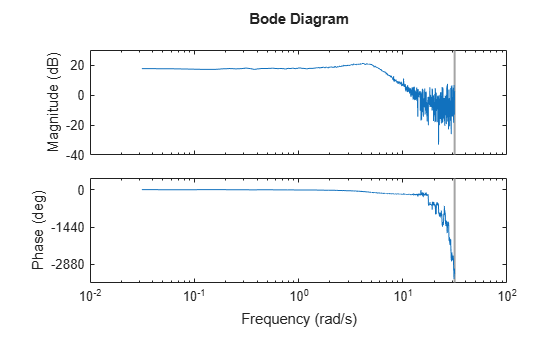`frdata` is a complex `idfrd` object with object properties that you can access using dot notation. For example, confirm the value of `Ts`.

`tsproperty = frdata.Ts`
```tsproperty = 0.1000 ```

You can also set property values. Set the `Name` property to `'DC_Converter'`.

`frdata.Name = 'DC_Converter';`

If you import `frdata` into the `System Identification` app, the app names this data `DC_Converter`, and not the variable name `frdata`.

Use `get` to obtain the full set of property settings.

`get(frdata)`
``` FrequencyUnit: 'rad/TimeUnit' Report: [1x1 idresults.frdest] SpectrumData: [] CovarianceData: [] NoiseCovariance: [] InterSample: {'zoh'} ResponseData: [1x1x1000 double] IODelay: 0 InputDelay: 0 OutputDelay: 0 Ts: 0.1000 TimeUnit: 'seconds' InputName: {''} InputUnit: {''} InputGroup: [1x1 struct] OutputName: {''} OutputUnit: {''} OutputGroup: [1x1 struct] Notes: [0x1 string] UserData: [] Name: 'DC_Converter' SamplingGrid: [1x1 struct] Frequency: [1000x1 double] ```

Convert a state-space model to a frequency response model using the `idfrd` command.

Load the data `z2` and estimate a second-order state-space model `sys`.

```load iddata2 z2 sys = ssest(z2,2);```

Convert `sys` to the `idfrd` model `frsys`.

`frsys = idfrd(sys)`
```frsys = IDFRD model. Contains Frequency Response Data for 1 output(s) and 1 input(s), and the spectra for disturbances at the outputs. Response data and disturbance spectra are available at 68 frequency points, ranging from 0.1 rad/s to 1000 rad/s. Output channels: 'y1' Input channels: 'u1' Status: Created by conversion from idss model. ```

Plot `frsys`.

`bode(frsys)`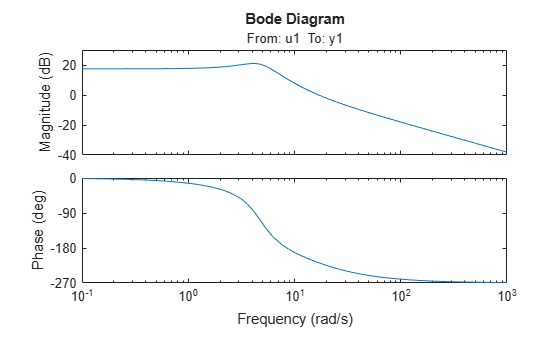`frsys` is an `idfrd` model that you can use as a dynamic system model or as estimation data for a time-domain or frequency-domain model.

Obtain the frequency response of a transfer function model and convert the response into an `idfrd` object.

Construct a transfer function model with one zero and three poles.

`systf = idtf([1 .2],[1 2 1 1]);`

Use `bode` to obtain the frequency response of `systf`, in terms of magnitude and phase, for the frequency vector `f`.

```f = logspace(-1,1,100); [mag,phase] = bode(systf,f);```

Use the values of `mag` and `phase` to compute the complex-valued response `response`.

`response = mag.*exp(1j*phase*pi/180);`

Create an `idfrd` object `frdata` to store `response`, specifying a sample rate `Ts` of 0.8.

```Ts = 0.8; frdata = idfrd(response,f,Ts)```
```frdata = IDFRD model. Contains Frequency Response Data for 1 output(s) and 1 input(s). Response data is available at 100 frequency points, ranging from 0.1 rad/s to 10 rad/s. Sample time: 0.8 seconds Status: Created by direct construction or transformation. Not estimated. ```

Plot the data.

`bode(frdata)`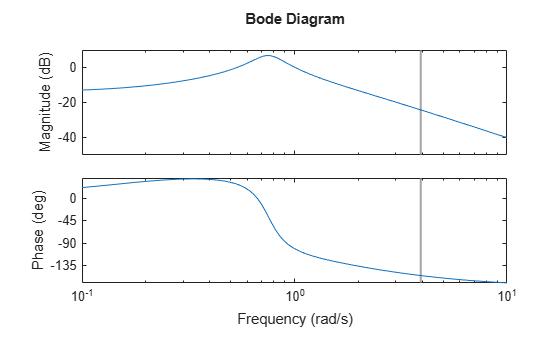`frdata` is a complex `idfrd` object.

Estimate a transfer function model from time-domain data and convert the resulting `idtf` model to an `idfrd` model. Estimate a new transfer function model from the frequency response data in the `idfrd` model. Compare the model responses with the original data.

Load time-domain data `z2` and use it to estimate a transfer function `sys` that has two poles and one zero.

```load iddata2 z2 sys = tfest(z2,2,1);```

Convert `sys` to an `idfrd` model and plot the frequency response.

```frsys = idfrd(sys); bode(sys)```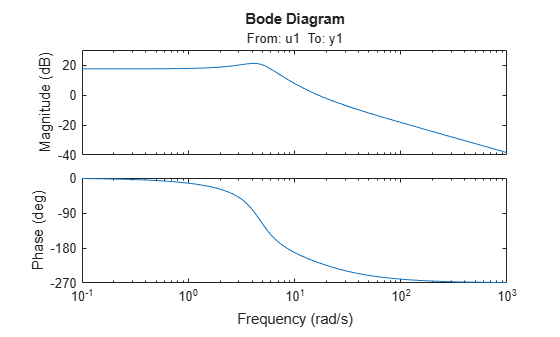Estimate a new transfer function `sys1` using the data from `frsys` as the estimation data.

`sys1 = tfest(frsys,2,1);`

Compare the responses of `sys` and `sys1` with the original estimation data `z2`.

`compare(z2,sys,sys1)`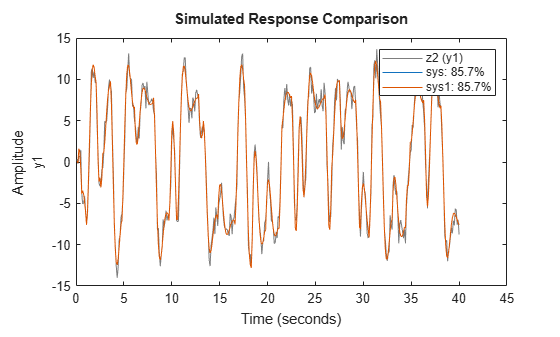The model responses are identical.

Introduced before R2006a

## Support평가판 신청